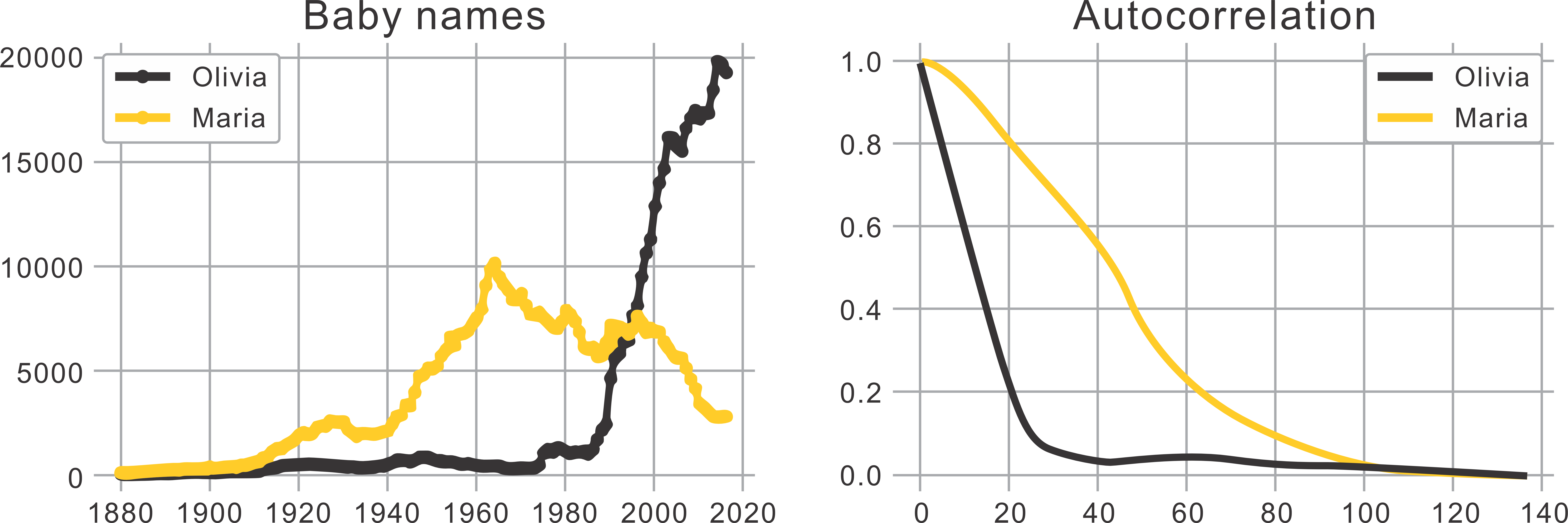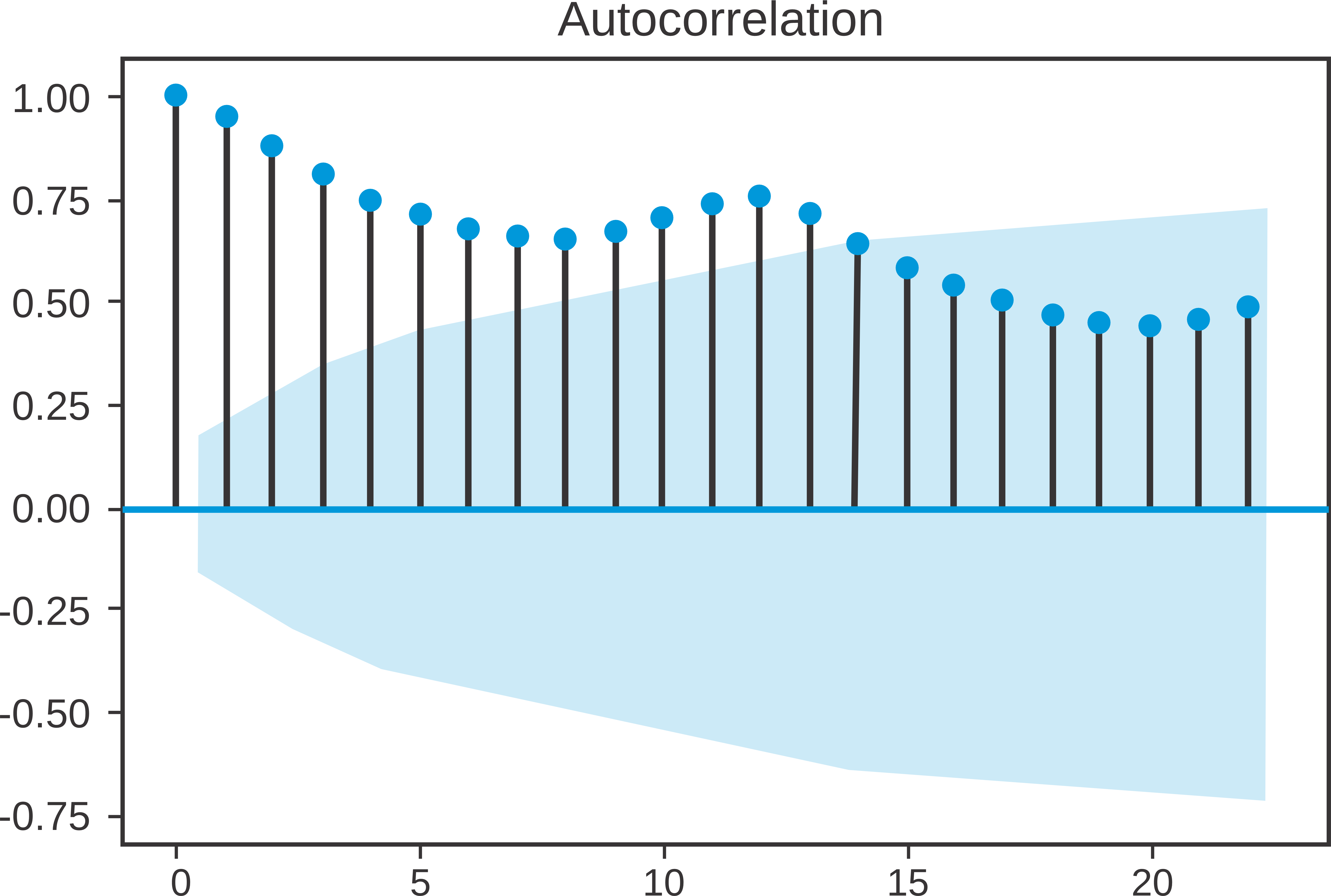Course Content

# Time Series Analysis

1. Time Series: Let's Start

2. Time Series Processing

3. Time Series Visualization

Time Series Analysis

##Autocorrelation

The next characteristic we will analyze is autocorrelation.

Autocorrelation measures how much future values in a time series depend linearly on past values. What examples can we give?The graph above shows the popularity of the names "Maria" and "Olivia" over 140 years. Olivia's autocorrelation decays much faster than Maria's: this can be explained by the fact that the popularity of the name Olivia was very low until 1980 and then increased very sharply. While the popularity of the name Maria did not have such sharp jumps and developed approximately the same over time.

Let's visualize the autocorrelation:Let's see how to interpret this chart. The graph shows the last 22 values from the dataset (they are shown as vertical lines). If these lines fall within the shaded blue area, this means that they do not have a significant correlation with the previous values.

As you can see on the graph, the first 13 values are correlated with the previous ones, while the next ones are not.

In summary, autocorrelation is useful for identifying statistically significant relationships between values in a time series.

Visualize the autocorrelation of the following dataset `air_quality_no2_long.csv` for 30 records.
1. Import the `plot_acf` function from `statsmodels.graphics.tsaplots`.
2. Visualize the autocorrelation for 30 `"value"` records of the `data` DataFrame.